# 计及新能源的微电网优化策略研究Research on New Energy Micro Grid Optimizing Strategy

• 全文下载: PDF(1182KB)    PP.119-129   DOI: 10.12677/SG.2019.93013
• 下载量: 234  浏览量: 420   科研立项经费支持

This paper considers wind, solar and storage in micro grid system. Firstly, the framework of micro grid system is analyzed and the mathematical models of each module in the system are established. Then the flexible load characteristics of adjustable load and translatable load are studied and modeled respectively. Finally, the NSGA2 multi-objective optimization algorithm is adopted to study micro grid operation cost and the service life of energy storage system as the objective function. An example is given to verify the feasibility and validity of the algorithm. Compared with conventional operation schemes, the method proposed in this paper can effectively reduce the operation cost of micro grid by optimizing load dispatch, and improve the service life of energy storage system to ensure the safe and economic operation of micro grid.

1. 引言

2. 风、光、储微电网系统的框架与模型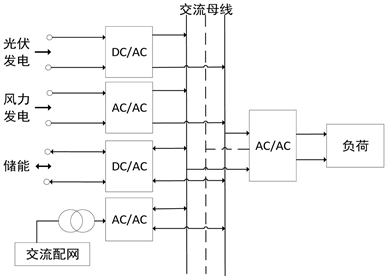Figure 1. Framework diagram of micro grid

2.1. 光伏发电出力模型

${P}_{PV}={Y}_{pv}×{f}_{pv}×\frac{\stackrel{¯}{{G}_{T}}\left[1+{\alpha }_{p}\left({T}_{c}-{T}_{c,STC}\right)\right]}{\stackrel{¯}{{G}_{T,STC}}}$ (1)

2.2. 风力发电出力模型

${p}^{w}=\left\{\begin{array}{l}0\text{\hspace{0.17em}}\text{\hspace{0.17em}}\text{\hspace{0.17em}}\text{\hspace{0.17em}}\text{\hspace{0.17em}}\text{\hspace{0.17em}}\text{\hspace{0.17em}}\text{\hspace{0.17em}}\text{\hspace{0.17em}}\text{\hspace{0.17em}}\text{\hspace{0.17em}}\text{\hspace{0.17em}}\text{\hspace{0.17em}}\text{\hspace{0.17em}}\text{\hspace{0.17em}}\text{\hspace{0.17em}}\text{\hspace{0.17em}}\text{\hspace{0.17em}}v<{v}_{in}或v>{v}_{out}\\ {p}_{r}^{w}\frac{v-{v}_{in}}{{v}_{r}-{v}_{in}}\text{\hspace{0.17em}}\text{\hspace{0.17em}}\text{\hspace{0.17em}}\text{\hspace{0.17em}}\text{\hspace{0.17em}}\text{\hspace{0.17em}}\text{\hspace{0.17em}}{v}_{in}\le v<{v}_{r}\\ {p}_{r}^{w}\text{\hspace{0.17em}}\text{\hspace{0.17em}}\text{\hspace{0.17em}}\text{\hspace{0.17em}}\text{\hspace{0.17em}}\text{\hspace{0.17em}}\text{\hspace{0.17em}}\text{\hspace{0.17em}}\text{\hspace{0.17em}}\text{\hspace{0.17em}}\text{\hspace{0.17em}}\text{\hspace{0.17em}}\text{\hspace{0.17em}}\text{\hspace{0.17em}}\text{\hspace{0.17em}}\text{\hspace{0.17em}}\text{\hspace{0.17em}}{v}_{r}\le v\le {v}_{out}\end{array}$ (2)

${P}_{W}\left\{{p}^{w}=0\right\}=1-\mathrm{exp}\left[-{\left(\frac{{v}_{in}}{c}\right)}^{k}\right]+\mathrm{exp}\left[-{\left(\frac{{v}_{out}}{c}\right)}^{k}\right]$ (3)

${P}_{W}\left\{{p}^{w}={p}_{r}^{w}\right\}=\mathrm{exp}\left[-{\left(\frac{{v}_{r}}{c}\right)}^{k}\right]-\mathrm{exp}\left[-{\left(\frac{{v}_{out}}{c}\right)}^{k}\right]$ (4)

${f}_{W}\left({p}^{w}\right)=\frac{kl{v}_{in}}{c}{\left(\frac{\left(1+\eta l\right){v}_{in}}{c}\right)}^{k-1}\mathrm{exp}\left[-{\left(\frac{\left(1+\eta l\right){v}_{in}}{c}\right)}^{k}\right]$ (5)

2.3. 风力发电出力模型

1) 蓄电池充电过程：

$E\left(t\right)-\left(1-\alpha \right)E\left(t-1\right)={P}_{c}\left(t\right){f}_{c}{T}_{c}$ (6)

2) 蓄电池放电过程

$E\left(t\right)+{P}_{f}\left(t\right){f}_{f}{T}_{f}=\left(1-\alpha \right)E\left(t-1\right)$ (7)

3) 储能电池的荷电状态(State Of Charge, SOC)：

$\text{SOC}=\frac{E\left(t\right)}{{E}_{\mathrm{max}}}$ (8)

4) 蓄电池组放电电量：

${E}_{c}=\underset{i=1}{\overset{T}{\sum }}{P}_{bi}\cdot \Delta {t}_{i}$ (9)

${{P}^{\prime }}_{bi}=\left\{\begin{array}{l}|{P}_{bi}|\text{}{P}_{bi}<0\\ \text{0}{P}_{bi}\ge \text{0}\end{array}$ (10)

3. 柔性负荷建模研究

3.1. 可调整负荷模型

${T}_{in}\left(t+1\right)={T}_{out}\left(t+1\right)-\left({T}_{out}\left(t+1\right)-{T}_{in}\left(t\right)\right){\text{e}}^{\frac{-\Delta t}{RC}}$ (11)

${T}_{in}\left(t+1\right)={T}_{out}\left(t+1\right)-1000\eta PR-\left({T}_{out}\left(t+1\right)-1000\eta PR-{T}_{in}\left(t\right)\right){\text{e}}^{\frac{-\Delta t}{RC}}$ (12)

${t}_{s}=RC\mathrm{ln}\frac{{T}_{\mathrm{min}}-{T}_{out}}{{T}_{\mathrm{max}}-{T}_{out}}$ (13)

${t}_{f}=RC\mathrm{ln}\frac{1000\eta R+{T}_{\mathrm{max}}-{T}_{out}}{1000\eta R+{T}_{\mathrm{min}}-{T}_{out}}$ (14)

${P}_{e}=\frac{{t}_{s}}{{t}_{s}+{t}_{f}}P$ (15)

3.2. 可平移负荷模型

${P}_{lk,i}=\left\{\begin{array}{l}{P}_{ek}\text{}{t}_{ak}\le i\le {t}_{ak}+{t}_{c}\\ 0\text{}其他\end{array}$ (16)

${P}_{l,i}=\underset{{k}_{1}=1}{\overset{{N}_{1}}{\sum }}{P}_{l{k}_{1},i}+\underset{{k}_{2}=1}{\overset{{N}_{2}}{\sum }}{P}_{l{k}_{2},i}$ (17)

4. 微电网多目标优化调度模型

4.1. 数据变量的采集

4.2. 微电网调度策略与假设

1) 对柔性负荷的调度策略与假设如下：假设本文研究的负荷(空调、电瓶充电装置、洗衣机)全部可调，根据预估的模拟数据，将空调设备设定的室内温度上下限，电瓶充电装置的工作起止时间、洗衣机的工作起止时间作为决策变量在满足约束条件的情况下进行调节，以满足目标函数的要求。

2) 微电网与交流配网的购电/售电关系如下：在风光出力不能够满足可平移负荷需求时，储能放电/微电网向交流配网购电，当风光出力过剩时，储能充电/微电网向交流配网售电。微电网与电网的购电/售电关系是通过多目标算法寻找最优化解集后自动形成的。

3) 本文认为微电网的运营商是风力发电系统、光伏发电系统、储能系统的投资主体，即风光模块发出的电能与储能模块存储的电能无需缴纳费用，但需要考虑设备运行成本，由于是日调度策略，因此本文不考虑投资成本。

4.3. 目标函数

4.3.1. 运行费用

$\begin{array}{l}\mathrm{min}{f}_{1}=\underset{i=1}{\overset{T}{\sum }}\left[{K}_{w\left(w\right)}{P}_{wi}+{K}_{w\left(pv\right)}{P}_{pvi}+{K}_{w\left(ba\right)}{P}_{bai}\right]\Delta {t}_{i}\\ \text{\hspace{0.17em}}\text{\hspace{0.17em}}\text{\hspace{0.17em}}\text{\hspace{0.17em}}\text{\hspace{0.17em}}\text{\hspace{0.17em}}\text{\hspace{0.17em}}\text{\hspace{0.17em}}\text{\hspace{0.17em}}\text{\hspace{0.17em}}\text{\hspace{0.17em}}\text{\hspace{0.17em}}+\underset{i=1}{\overset{T}{\sum }}\left[{C}_{in,i}\cdot \left({P}_{in,i}\cdot \Delta {t}_{i}\right)-{C}_{out,i}\cdot \left({P}_{out,i}\cdot \Delta {t}_{i}\right)\right]\end{array}$ (18)

4.3.2. 蓄电池组使用寿命

$\mathrm{min}{E}_{c}=\underset{i=1}{\overset{T}{\sum }}{{P}^{\prime }}_{bi}\cdot \Delta {t}_{i}$ (19)

4.4. 约束条件

1) 各组件功率平衡约束

${P}_{pvi}{\eta }_{DC/AC}+{P}_{wi}{\eta }_{AC/AC}=\frac{{P}_{e,i}}{{\eta }_{AC/AC}}+\frac{{P}_{l,i}}{{\eta }_{AC/AC}}+\frac{{P}_{ba,i}}{{\eta }_{DC/AC}}+\frac{{P}_{out,i}}{{\eta }_{AC/AC}}$ (20)

${P}_{pvi}{\eta }_{DC/AC}+{P}_{wi}{\eta }_{AC/AC}+{P}_{ba,i}{\eta }_{DC/AC}+{P}_{in,i}{\eta }_{AC/AC}=\frac{{P}_{e,i}}{{\eta }_{AC/AC}}+\frac{{P}_{l,i}}{{\eta }_{AC/AC}}$ (21)

2) 空调温度范围约束

${T}_{in,\mathrm{min}}\le {T}_{in}\left(t\right)\le {T}_{in,\mathrm{max}}$ (22)

3) 可平移负荷约束

$\begin{array}{l}{t}_{m}\le {t}_{ak}\\ {t}_{ak}+{t}_{c}\le {t}_{n}\end{array}$ (23)

$19:00\le {t}_{ak}\le {t}_{ak}+{t}_{c}\le 次日10:00$ (24)

${t}_{ak}-4:00\le {t}_{ak}\le {t}_{ak}+{t}_{c}\le {t}_{ak}+4:00$ (25)

4) 储能约束

$|{P}_{bi}|\le {P}_{bn}{\eta }_{dd}$ (26)

$10%\le {\text{SOC}}_{i}\le 100%$ (27)

5) 并网约束

$\begin{array}{l}{P}_{in,i}\le {P}_{an}{\eta }_{ad}\\ {P}_{out,i}\le {P}_{an}/{\eta }_{ad}\end{array}$ (28)

5. 模型求解与算例分析

5.1. 系统流程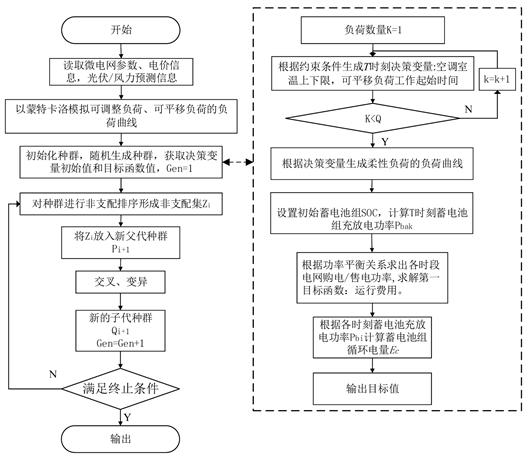Figure 2. System flow chart

5.2. 算例分析

5.2.1. 参数设定Table 1. Price of purchasing and selling electricity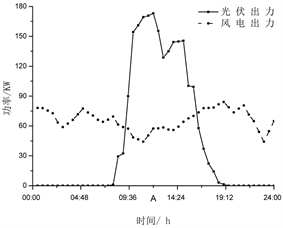Figure 3. Wind power and photovoltaic output curve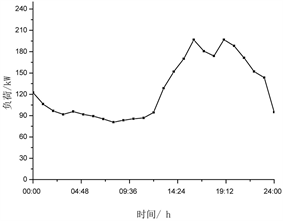5.2.2. 优化结果分析Figure 5. Pareto curve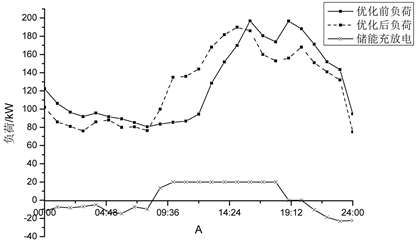Figure 6. Flexible load optimization and energy storage charge and discharge scheduling

5.2.3. 优化前后的对比分析Table 2. Comparisons between optimal scheduling scheme and conventional scheduling scheme

6. 结论

  董庆远, 潘曙光, 刘香. 带储能系统的用电负荷实时调控及其控制器研究[J]. 电器与能效管理技术, 2018(2): 60-63.  玄京岩, 张艳, 金成日, 等. 分布式光伏电源接入容量对主动配电网的影响研究[J]. 电器与能效管理技术, 2018(1): 25-28.  佚名. 促进风电消纳的配电网分布式电源与电动汽车充电站联合鲁棒规划[J]. 可再生能源, 2018, 36(11): 62-68.  于芃, 刘兴华, 孙树敏, 等. 高可再生能源渗透率海岛微电网运行控制[J]. 电网技术, 2018, 42(3): 779-788.  彭寒梅, 郭颖聪, 昌玲, 等. 基于系统短期时序状态转移抽样法的孤岛运行微电网可靠性评估[J]. 电工电能新技术, 2018, 37(1): 66-74.  国家发展改革委. 国家重点节能低碳技术推广目录(摘录) [J]. 有色冶金节能, 2015, 31(2): 1-5.  Zare, M., Niknam, T., Azizi-panah-Abarghooee, R., et al. (2016) Newstochastic Bi-Objective Optimal Cost and Chance of Operation Management Approach for Smart Microgrid. IEEE Transactions on Industrial Informatics, 12, 2031-2040. https://doi.org/10.1109/TII.2016.2585379  张忠, 王建学, 曹晓宇. 基于负荷分类调度的孤岛型微网能量管理方法[J]. 电力系统自动化, 2015(15): 17-23, 109.  Bracale, A., Caramia, P., Carpinelli, G., et al. (2015) Optimal Control Strategy of a DC Micro Grid. International Journal of Electrical Power & Energy Systems, 67, 25-38. https://doi.org/10.1016/j.ijepes.2014.11.003  王江波, 费标青, 王越, 等. 户用微电网能量调度日前计划研究[J]. 电测与仪表, 2013, 50(8): 81-86.  Logenthiran, T., Srinivasan, D., Khambadkone, A.M., et al. (2012) Multiagent System for Real-Time Operation of a Microgrid in Real-Time Digital Simulator. IEEE Transactions on Smart Grid, 3, 925-933. https://doi.org/10.1109/TSG.2012.2189028  金鑫, 袁越, 傅质馨, 等. 天气类型聚类的支持向量机在光伏系统输出功率预测中的应用[J]. 现代电力, 2013, 30(4): 42-49.  林卫星, 文劲宇, 艾小猛, 等. 风电功率波动特性的概率分布研究[J]. 中国电机工程学报, 2012, 32(1): 38-46.